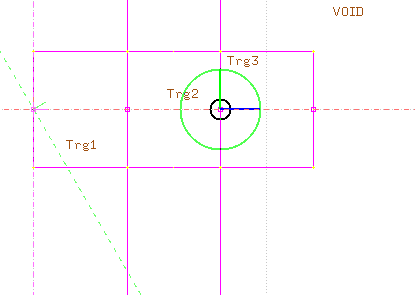# Number conservation problem

Dear Fluka experts,
Here I have a problem regarding number conservation.Consv2.flair (1.9 KB) . All the three targets are vacuum.
Numbers i got after running the problem using USRBDX
Tot. resp. (Part/pr)
Trg1 to trg 2 =5.186E-04 +/- 1.444615 %
Void to trg2= Nil
trg3 to trg2 =Nil
trg2 to trg1= Nil
trg2 to trg3=4.524E-4
All above are as expected as per geometry and distance from isotropic source.
But the below is confusing
trg2 to void= 1.4856970E-03 +/- 4.654177 %

trg2 to outside void numbers are too high. More than what has come inside.
Please guide me where I have gone wrong.
Consv2_25_sum.lis (24.2 KB)

Dear Sunil,

these results may seem counter intuitive, but they are easily explained based on how fluence is scored in FLUKA with the USRBDX scoring:

Imagine a surface (S) having an infinitesimal thickness dt. When a particle is passing through this surface with an angle \theta with respect to the normal vector of the surface, the path length in the surface will be dt / \cos \theta.

So the average fluence (\Phi) in the surface will be the sum of these infinitesimal track lengths divided by the volume S \cdot dt.

\Phi = \lim_{dt\to0} \frac{\sum_{i} \frac{dt}{\cos \theta_{i}}} {S \cdot dt}

In your scoring the the angles of particles exiting Trg2 to the VOID are close to 90 degrees, thus giving a much bigger contribution to the fluence than particles crossing a surface close to perpendicular to it.

With a USRBDX scoring there is an option to score the current (J) of particles, which is simply the number of particles crossing the surface

J = \frac{dN}{dS}

Cheers,
Dávid

1 Like

Thank you sir
I should see USRBDX carefully when such areas whose normal are near 90 degrees to particles. If surface areas increase this result also increases this I could see.